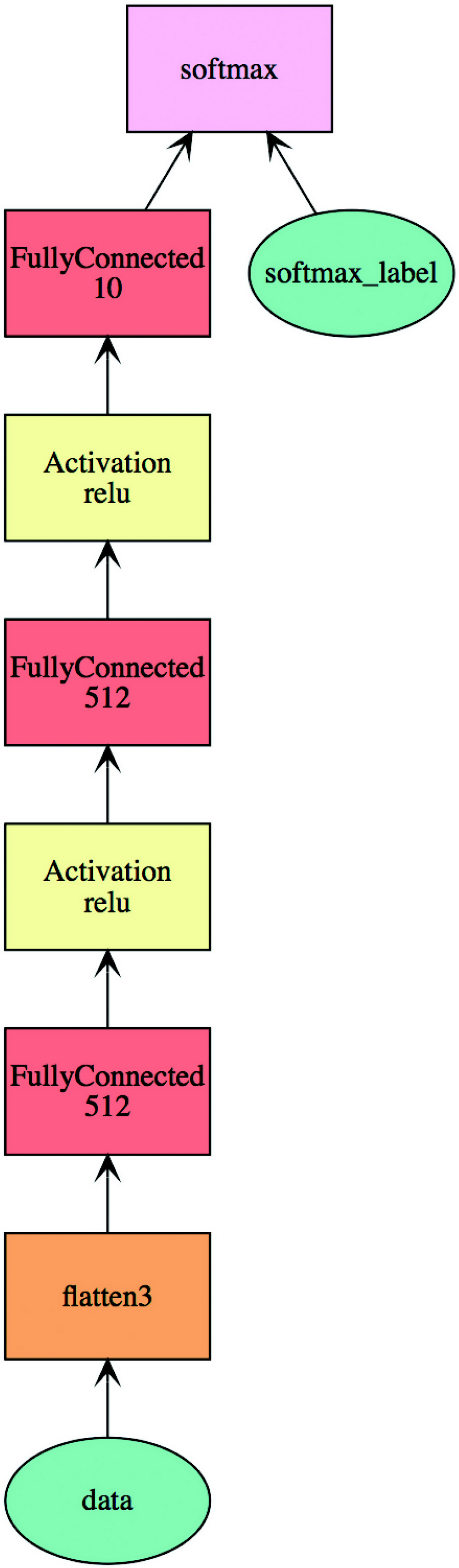# AI 安全之对抗样本入门 (36)：打造对抗样本工具箱 3.5(MXNet)

MXNet 是亚马逊开发的深度学习库，它拥有类似于 Theano 和 TensorFlow 的数据流图，并且可以在常见的硬件平台上运行。MXNet 还提供了 R、C++、Scala 等语言的接口。我们以解决经典的手写数字识别的问题为例，介绍 MXNet 的基本使用方法，代码路径为：

https://github.com/duoergun0729/adversarial_examples/blob/master/code/2-mxnet.ipynb
1. 加载相关库

import mxnet as mximport logging
1. 加载数据集

MXNet 中也针对常见的数据集进行了封装，免去了用户手工下载的过程并简化了预处理的过程：

mnist = mx.test_utils.get_mnist()batch_size = 128train_iter = mx.io.NDArrayIter(mnist['train_data'], mnist['train_label'],batch_size, shuffle=True)val_iter = mx.io.NDArrayIter(mnist['test_data'], mnist['test_label'],batch_size)
1. 定义网络结构

data = mx.sym.var('data')data = mx.sym.flatten(data=data)#全连接fc1  = mx.sym.FullyConnected(data=data, num_hidden = 512)act1 = mx.sym.Activation(data=fc1, act_type="relu")fc2  = mx.sym.FullyConnected(data=act1, num_hidden = 512)act2 = mx.sym.Activation(data=fc2, act_type="relu")fc3  = mx.sym.FullyConnected(data=act2, num_hidden=10)# softmax 输出mlp  = mx.sym.SoftmaxOutput(data=fc3, name='softmax')mlp_model = mx.mod.Module(symbol=mlp, context=ctx)

import matplotlib.pyplot as pltmx.viz.plot_network(mlp).view()1. 定义损失函数和优化器

1. 训练与验证

MXNet 的训练和验证过程是分开的，训练阶段加载优化器的配置，可以指定每训练 100 个批次，打印中间结果。

mlp_model.fit(train_iter,              eval_data=val_iter,              optimizer='sgd',              optimizer_params={'learning_rate':0.1},              eval_metric='acc',              batch_end_callback = mx.callback.Speedometer(batch_size, 100),              num_epoch=20)

test_iter = mx.io.NDArrayIter(mnist['test_data'], mnist['test_label'], batch_size)acc = mx.metric.Accuracy()mlp_model.score(test_iter, acc)print(acc)

INFO:root:Epoch Train-accuracy=0.996438INFO:root:Epoch Time cost=4.017 INFO:root:Epoch Validation-accuracy=0.976167EvalMetric: {'accuracy': 0.9761669303797469}

MXNet 保存的模型文件后缀为 parms。

mlp_model.save_checkpoint('models/mxnet.parms',20)